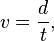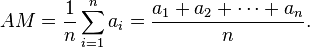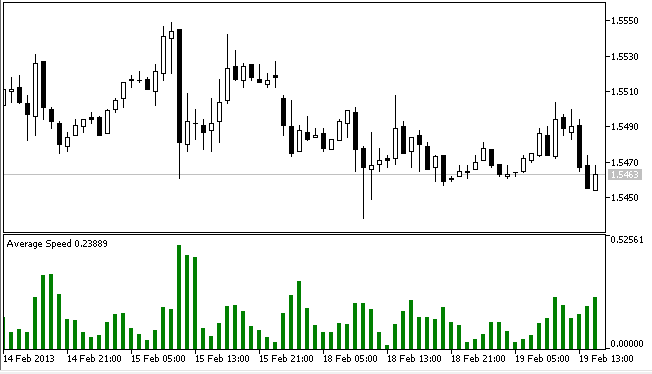Interesting script?
So post a link to it -
let others appraise it

You liked the script? Try it in the MetaTrader 5 terminal# Average Speed - indicator for MetaTrader 5

Views:
13585
Rating:
Published:
2013.02.19 18:03
Updated:
2013.02.25 09:25

The indicator's output is calculated base on mathematical approach.

Calculate Price Speed:

Galileo defined speed as the distance covered per unit of time. (http://en.wikipedia.org/wiki/Speed)

Equation:where v is speed, d is distance and t is time. In this indicator we will define d as distance between price series and t is in minute. We use minute instead of second because second is to fast while sometime we found no price movement for minutes. This could be annoying for some peoples.

Calculate Average Speed of Price:

Indicator calculate Average Speed using Arithmetical  Mean. (http://en.wikipedia.org/wiki/Average, http://en.wikipedia.org/wiki/Arithmetical_mean)

Equation:

If n numbers are given, each number denoted by ai, where i = 1, ..., n, the arithmetic mean is the [sum] of the ai's divided by n orin this indicator n is days, a is speed and AM is output of indicator buffer calculated in point/minute.OneSideGaussianMA

The Moving Average drawn on the basis of Gauss algorithmThe asymmetry indicator

The indicator which defines the moments when the market becomes asymmetric and there are strong trend changesTrendPower

The trend indicator drawn on the basis of a comparison of six levels of simple Moving Averages with High and Low of the current candlestickFX_Sniper_Ergodic_CCI

The ergodic CCI.